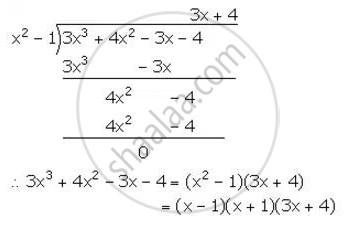Share

# The Polynomial Px3 + 4x2 – 3x + Q is Completely Divisible by X2 – 1; Find the Values of P and Q. Also, for These Values of P and Q Factorize the Given Polynomial Completely. - Mathematics

Course

#### Question

The polynomial px3 + 4x2 – 3x + q is completely divisible by x2 – 1; find the values of p and q. Also, for these values of p and q factorize the given polynomial completely.

#### Solution

Let f(x) = px3 + 4x2 – 3x + q
It is given that f(x) is completely divisible by (x2 – 1) = (x + 1)(x – 1).
Therefore, f(1) = 0 and f(-1) = 0
f(1) = p(1)3 + 4(1)2 – 3(1) + q = 0
p + q + 1 = 0 …(i)
f(-1) = p(-1)3 + 4(-1)2 – 3(-1) + q = 0
-p + q + 7 = 0 …(ii)
Adding (i) and (ii), we get,
2q + 8 = 0
q = -4
Substituting the value of q in (i), we get,
p = -q – 1 = 4 – 1 = 3
∴ f(x) = 3x3 + 4x2 – 3x – 4
Given that f(x) is completely divisible by (x2 – 1).Is there an error in this question or solution?